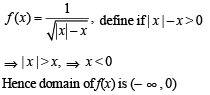Courses

# Test: 35 Year JEE Previous Year Questions: Functions

## 17 Questions MCQ Test Maths 35 Years JEE Mains & Advance Past year Papers Class 12 | Test: 35 Year JEE Previous Year Questions: Functions

Description
This mock test of Test: 35 Year JEE Previous Year Questions: Functions for JEE helps you for every JEE entrance exam. This contains 17 Multiple Choice Questions for JEE Test: 35 Year JEE Previous Year Questions: Functions (mcq) to study with solutions a complete question bank. The solved questions answers in this Test: 35 Year JEE Previous Year Questions: Functions quiz give you a good mix of easy questions and tough questions. JEE students definitely take this Test: 35 Year JEE Previous Year Questions: Functions exercise for a better result in the exam. You can find other Test: 35 Year JEE Previous Year Questions: Functions extra questions, long questions & short questions for JEE on EduRev as well by searching above.
QUESTION: 1

### The domain of sin-1 [log3 (x/3)] is

Solution:

Domain of sin-1x is [-1,1]

Therefore, -1 ≤ log3(x/3) ≤ 1

3-1 ≤ x/3 ≤ 3

1 ≤ x ≤ 9

Therefore, the domain of sin-1[log3(x/3)] is [1,9]

QUESTION: 2

### The function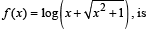Solution: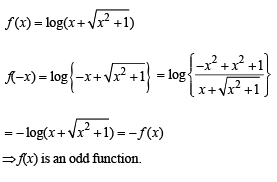QUESTION: 3

### Domain of definiti on of the function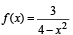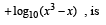Solution: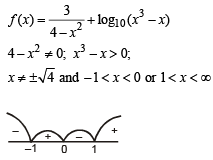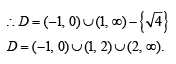QUESTION: 4

If f : R → R satisfies f (x + y) = f ( x) + f (y) , for all  x,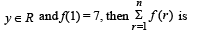Solution: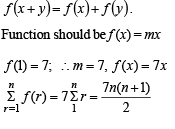QUESTION: 5

A function f from the set of  natural numbers to integers defined by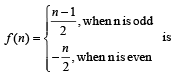Solution:

We have f: N →I
If x and y are two even natural numbers,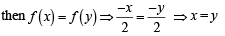Again if x and y are two odd natural numbers then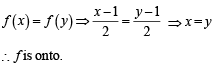Also each negative integer is an image of even natural number and each positive integer is an image of odd natural number.

∴ f is onto.
Hence f is one one and onto both.

QUESTION: 6

The range of the function f (x) = 7- x Px -3 is

Solution: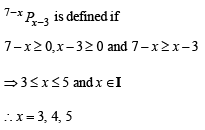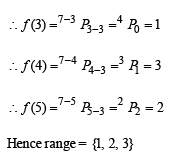QUESTION: 7

If f : R → S, defined by

f (x) = sin x - √3 cosx+ 1, is onto, then the interval of S is

Solution:

f (x) is onto ∴ S = range of f (x)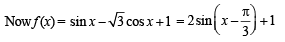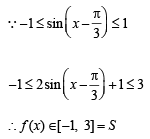QUESTION: 8

The graph of the function y = f(x) is symmetrical about the line x = 2, then

Solution:

Let us consider a graph symm. with respect to line x = 2 as shown in the figure.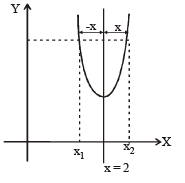From the figure

f (x1) = f (x2), where x1 = 2-x and x2 = 2+x
∴ f (2 - x) = f (2+x)

QUESTION: 9

The domain of the function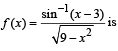Solution: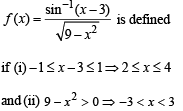Taking common solution of  (i) and (ii), we get 2 < x < 3  ∴ Domain = [2, 3)

QUESTION: 10

L et f : (– 1, 1) → B , be a function defined by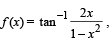then f is both one - one and onto when B is the interval

Solution: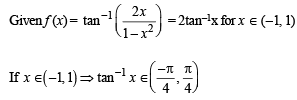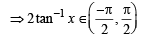Clearly, range of f (x)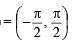For f to be onto, codomain = range
∴ Co-domain of function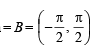QUESTION: 11

A function is matched below against an interval where it is supposed to be increasing. Which of the following pairs is incorrectly matched?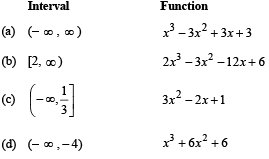Solution:

Clearly function f (x) = 3x2 - 2x+1 is increasing when
f ' (x) = 6x – 2 > 0 ⇒ x ∈[1 / 3,∞)

∴ f (x) is incorrectly matched with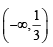QUESTION: 12

A real valued function f(x) satisfies the functional equation f(x – y) = f(x) f(y) – f(a – x) f(a + y) where a is a given constant and f(0) = 1, f(2a – x) is equal to

Solution:

f(2a – x) = f (a – (x – a))
= f(a) f(x – a) – f(0) f(x) = f(a) f(x –a) – f(x)
= – f(x)
[∴ x = 0, y = 0, f(0) = f2(0)- f2(a)
⇒ f2(a) = 0
⇒ f(a) = 0]
⇒ f(2a - x) = - f(x)

QUESTION: 13

The largest interval lying in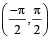for which the function,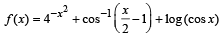, is defined, is

Solution: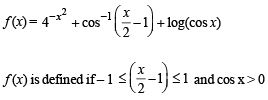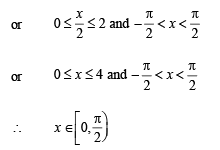QUESTION: 14

Let f: N→Y be a function defined as f(x) = 4x + 3 where Y = {y ∈ N : y = 4x + 3 for some x ∈ N}.

Show that f is invertible and its inverse is

Solution:

Clearly f is one one and onto, so invertible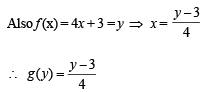QUESTION: 15

Let f(x) = ( x + 1)2 – 1,x > –1

Statement -1 : The set {x : f(x) = f –1(x) = {0, –1}

Statement-2 : f is a bijection.

Solution:

Given that f (x)  = (x + 1)2 –1,  x > –1 Clearly Df = [–, ∞) but co-demain is not given.
Therefore  f (x) need not be necessarily onto.
But if f (x) is onto then as f (x) is one one also, (x + 1) being something +ve, f–1(x) will exist where (x + 1)2 –1 = y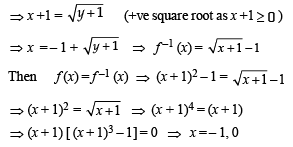∴ The statement-1 is correct but statement-2 is false.

QUESTION: 16

For real x, let f (x) = x3 + 5x + 1, then

Solution:

Given that f (x) = x3 + 5x + 1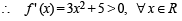⇒ f (x) is strictly increasing on R
⇒ f (x) is one one
∴ Being a polynomial f (x) is cont. and inc.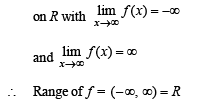Hence f is onto also. So, f is one one and onto R.

QUESTION: 17

The domain of the function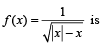Solution: